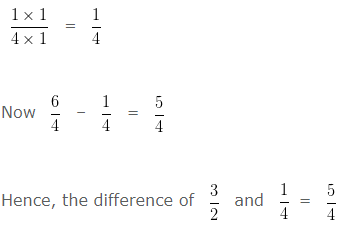# Fraction For Class 4

Fraction For Class 4, deals with various concepts which are as under:-

• Proper Fraction | Improper Fraction | Mixed Fraction
• Like Fractions | Unlike Fractions
• Fraction in Simplest Form
• Equivalent Fraction
• Conversion of Mixed Fraction into an Improper Fraction
• Conversion of Improper Fraction into Mixed Fraction
• Comparing Fractions
• Addition of Like Fractions and Unlike Fractions
• Subtraction of Like Fractions or Unlike Fractions

#### Fraction For Class 4 – Proper Fraction | Improper Fraction | Mixed Fraction

Proper Fraction

Proper Fraction is a Fraction whose numerator is less than its denominator.

i.e Numerator < Denominator

For Example – 1/3, 3/7, 5/6 are Proper Fractions.

Improper Fraction

Improper Fraction is a Fraction whose numerator is greater than or equal to its denominator.

i.e Numerator ≥ Denominator

For Example – 3/2, 7/4, 6/5 are Improper Fractions.

Mixed Fraction

Mixed Fraction is a Fraction having a combination of a Whole number or a Proper Fraction.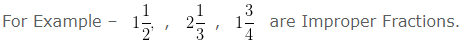Question 1

Is 12/7 an Improper Fraction?

Explanation :

In the given Fraction, Numerator = 12
The Denominator = 7
Here, 12 > 7
i.e, Numerator > Denominator
Hence, 12/7 is an Improper Fraction

Question 2

Is 3/4 an Improper Fraction?

Explanation :

In the given Fraction, Numerator = 3
The Denominator = 4
Here, 3 < 4
i.e, Numerator < Denominator
Hence, 3/4 is not an Improper Fraction

Question 3

Is 3/8 a Proper Fraction?

Explanation :

In the given Fraction, Numerator = 3
The Denominator = 8
Here 3 < 8
i.e, Numerator < Denominator
Hence, 3/8 is a Proper Fraction.

Question 4

Is 5/4 a Proper Fraction?

Explanation :

In the given Fraction, Numerator = 5
The Denominator = 4
Here 5 > 4
i.e, Numerator > Denominator
Hence, 5/4 is not a Proper Fraction.

#### Fraction For Class 4 – Like Fractions | Unlike Fractions

Like Fractions

Fractions having the same denominators are called Like Fractions.

For example 1/2, 3/2 and 5/2 are Like Fractions.

Unlike Fractions

Fractions having different denominators are called Unlike Fractions.

For example 2/3, 3/5 and 4/7 are Unlike Fractions.

Question 5

Are the following Fractions are Like Fractions or Unlike Fractions?

2/3, 4/3 and 5/3

Explanation :

In case of Like Fraction, the denominator of the Fractions are the same.

While, In case of Unlike Fraction denominator of the Fractions are not same.

In the present case, the given Fractions are

2/3, 4/3 and 5/3

Since, the Denominator in the present cases is 3 , which is the same for all Fractions, the given Fractions are Like Fractions

Question 6

Are the following Fractions are Like Fractions or Unlike Fractions?

5/6, 2/3 and 4/5

Explanation :

In case of Like Fraction, the denominator of the Fractions are the same.

While, In case of Unlike Fraction denominator of the Fractions are different.

In the present case, the given Fractions are

5/6, 2/3 and 4/5

Since the Denominator in the present cases are 6 , 3 , 5 , which are not same, the given Fractions are Unlike Fractions

#### Fraction For Class 4 – Fraction in Simplest Form

Fraction in Simplest Form – A Fraction is said to be in simplest form when the numerator and denominator of a fraction has only common factor i.e, 1

In other words, To simplify a fraction in simplest form we divide the numerator and denominator of a fraction by their H.C.F ( Highest Common Factor ).

Question 7

Convert  15/45  into its Simplest Form?

Explanation :

Finding HCF of 15 and 45.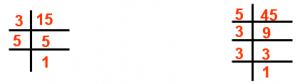Factors of 15 are  35

Factors of 45 are  53,  3

HCF of 15 and 45 = 3 x 5 = 15

Divide Numerator and Denominator by their HCF i.e, 15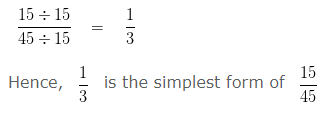#### Equivalent Fraction

Equivalent Fractions are Fractions that represent the same amount or same Fractions.

Question 8

Check whether 2/3 and 6/9 are Equivalent Fractions ?

Explanation :

If two fractions are equivalent, on cross multiplication, their Products will be the same

Cross multiplying 2/3 and 6/9

we get,

2 x 9 = 18

and

6 x 3 = 18

Since, the products are same

Hence, 2/3 and 6/9 are Equivalent Fractions.

Question 9

Check whether 5/9 and 10/16 are Equivalent Fractions ?

Explanation :

If two fractions are equivalent, on cross multiplication, their Products will be the same

Cross multiplying 5/9 and 10/16

We get,

5 x 16 = 80

and

10 x 9 = 90

Since, the products are not same

Hence, 5/9 and 10/16 are not Equivalent Fractions.

#### Finding Equivalent Fraction with given Numerator

Question 10

Write an equivalent Fraction of 2/3 with Numerator 12 .

Explanation :

Let,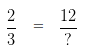In order to obtain an equivalent fraction, we need to multiply the Numerator and Denominator of a given number , by same digit

To get 12 as Numerator, we have to multiply 2 by 6

In order to get an equivalent Fraction, we have to multiply the Denominator by the same number, i.e, 6
i.e,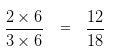#### Finding Equivalent Fraction with given Denominator

Question 11

Write an equivalent Fraction of 15/12 with denominator 4 .

Explanation :

Let,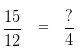In order to obtain an equivalent fraction, we need to divide the Numerator and Denominator of a given number , by same digit

To get 4 in the denominator, we need to divide 12 by 3

So, in order to get an equivalent Fraction, we have to divide the Numerator also by 3

i.e,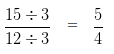Hence, 5/4 is an equivalent Fraction of 15/12 with denominator 4

#### Fraction For Class 4 – Conversion of Mixed Fraction into an Improper Fraction

To Convert Mixed Fraction into Improper Fraction, we follow the steps given below:-

Step 1: Multiply the Whole Number Part and Denominator of the Mixed Fraction

Step 2: Add the Numerator to the product obtained in Step 1

Step 3: Write the sum as the numerator and denominator would remain the same, as in the Mixed Fraction.

Question 12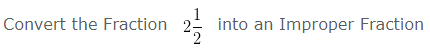Explanation :

A combination of Whole Number and a Proper Fraction is called a Mixed Fraction
Here,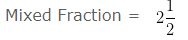Whole Number Part = 2
Numerator = 1
Denominator = 2
Step 1 : Multiply the Whole Number Part and Denominator of the Mixed Fraction
2 x 2 = 4
Step 2 : Add the Numerator to the product obtained in Step 1
1 + 4 = 5
Write the sum as the numerator and denominator would remain the same , as in the Mixed Fraction.
5/2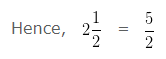#### Fraction For Class 4 – Conversion of Improper Fraction into Mixed Fraction

To Convert Improper Fraction into Mixed Fraction, we follow the steps given below:-

Step I: Divide the Numerator by the Denominator.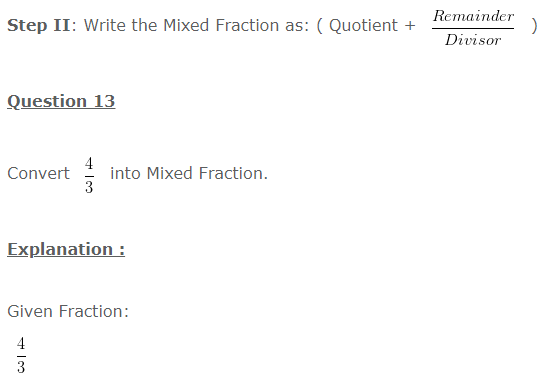Divide the Numerator by the Denominator
On dividing 4 by 3
We get Quotient = 1
Remainder = 1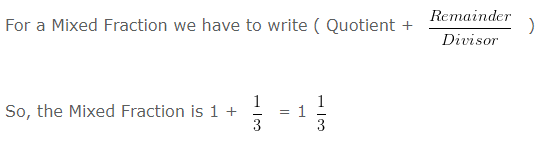#### Fraction For Class 4 – Comparing Fractions

Comparing Fractions can be divided in two categories:

• Comparison of Like Fractions
• Comparison of Unlike Fractions
First Method : By Converting Given Fractions into Like Fractions
Second Method : By Cross Multiplication Method

#### COMPARISION OF LIKE FRACTION

Question 14

Compare 7/3 and 4/3

Explanation :

7/3 and 4/3 are Like Fraction as Denominator of both the Fractions is same i.e, 3

In case of Like Fraction, the Fraction with the higher numerator is Higher

On Comparing the Numerators, we find

7 > 4

Hence, 7/3 > 4/3

#### COMPARISON OF UNLIKE FRACTION

Question 15

Compare 2/3 and 1/2

Explanation :

2/3 and 1/2 are Unlike Fractions as Denominator of both the Fractions is different i.e, 3 and 2

To compare Unlike Fractions we have two methods:

First Method : By Converting Given Fractions into Like Fractions

Given Fractions :

2/3 and 1/2

Taking LCM of the Denominator of both the Fractions.

i.e, LCM of 3 and 2 is 6

To change the Unlike Fractions into Like Fractions

We have to multiply the Numerator and Denominator of the Given Fraction by a number such that the Denominator of the given Fraction becomes equal to its LCM i.e, 6

We have to multiply Numerator and Denominator of  2/3 by 2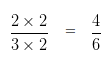We have to multiply Numerator and Denominator of 1/2 by 3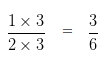Now the Given Fractions are Like Fraction as their Denominator become same.

On Comparing their Numerators, we find

4 > 3

Therefore, 4/6 > 3/6

Hence, 2/3 > 1/2

Second Method : By Cross Multiplication Method

On Cross multiplying 2/3 and 1/2

In Cross Multiplication Method we multiply the Numerator of First Fraction namely 2 by the Denominator of Second Fraction namely 2 i.e,

2 x 2 = 4

Then, we multiply the Numerator of Second Fraction namely 1 by the Denominator of First Fraction namely 3 i.e,

1 x 3 = 3

Since, 4 > 3

Therefore,  2/3 > 1/2

#### Fraction For Class 4 – Addition of Like Fractions and Unlike Fractions

To add Like Fractions, we add the numerators and write the sum over the same denominator.

To add Unlike Fractions, first we have to change the Unlike Fractions, into equivalent Like Fractions, and then add the two equivalent Like Fractions

Example on Addition of Like Fractions

Question 16

Find the sum of 9/14 and 3/14 and reduce to its lowest term.

Explanation :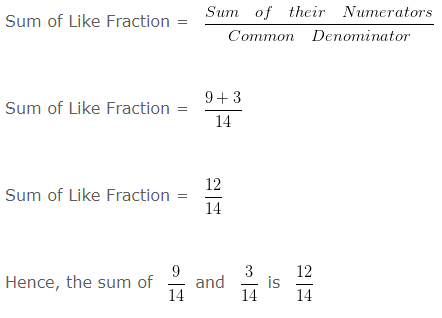Simplifying further,
HCF of 12 and 14 is 2
Dividing both Numerator and Denominator by their HCF i.e,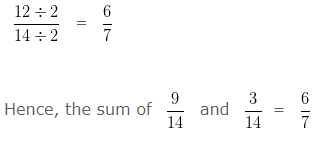Example on Addition of Unlike Fractions

Question 17

Find the sum of 1/3 and 2/4 and reduce to its lowest term.

Explanation :

In order to add Unlike Fraction
Firstly, we have to change the Unlike Fraction, into equivalent Like Fractions, and then add the two equivalent Like Fractions
Take LCM of 3 and 4 is 12
Converting the Unlike Fraction into Equivalent Like Fraction
We have to multiply , both the numerator and denominator of 1/3 by 4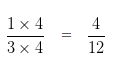We have to multiply , both the numerator and denominator of 2/4 by 3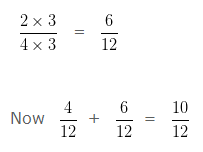Simplifying further,
HCF of 10 and 12 is 2
Dividing both Numerator and Denominator by their HCF i.e,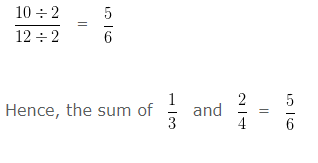#### Fraction For Class 4 – Subtraction of Like Fractions or Unlike Fractions

To subtracts Like Fractions, we subtract the numerators and write the difference over the same denominator.

To subtracts Unlike Fractions, first we have to change the Unlike Fractions, into equivalent Like Fractions, and then subtract the two equivalent Like Fractions

Example on Subtraction of Like Fractions

Question 18

Find the difference of 7/8 and 3/8 and reduce to its lowest term.

Explanation :Hence, the difference of 7/8 and 3/8 = 1/2

Example on Subtraction of Unlike Fractions

Question 19

Find the difference of 3/2 and 1/4 and reduce to its lowest term.

Explanation :

To find the difference of Unlike Fraction
Firstly, we have to change the Unlike Fraction into equivalent Like Fraction, and then subtract the two equivalent Like Fraction
Take LCM of 2 and 4 is 4
Converting the Unlike Fraction into Equivalent Like Fraction
We have to multiply Numerator and Denominator of 3/2 by 2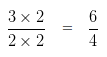We have to multiply Numerator and Denominator of 1/4 by 1